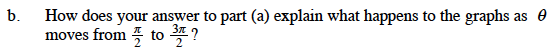### Home > CALC > Chapter Ch10 > Lesson 10.3.2 > Problem10-128

10-128.
1. For the three polar curves r1 = 2 + cos θ, r2 = 2 + 2 cos θ, and r3 = 2 + 3 cos θ: Homework Help ✎

1. What are the minimum values of r for each of the functions? What is the corresponding θ-value?

2. How does your answer to part (a) explain what happens to the graphs as θ moves from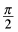to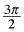?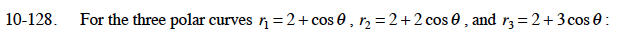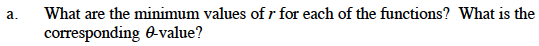Recall that –1 ≤ cos(θ) ≤ 1.